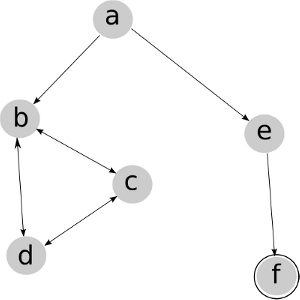Practice Exercise 3.B
Uninformed Search

## 2: Learning Goals

• Apply basic properties of search algorithms: completeness, optimality, time and space complexity of search algorithms.
• Select the most appropriate search algorithms for specific problems.

## 3: Directed Questions

1. What is meant by search algorithm completeness? [solution]

2. What is meant by search algorithm optimality? [solution]

3. What are the advantages of breadth-first search (BFS) over depth-first search (DFS)? [solution]

4. What is the advantage of DFS over BFS? [solution]

## 4: Exercise: Uninformed Search

1. Consider the search problem represented in the following figure, where a is the start node and f is the goal node. Would you prefer DFS or BFS for this problem? Why? [solution]2. Which sequences of paths are explored by BFS and DFS in this problem? [solution]

## 5: Learning Goals Revisited

• Apply basic properties of search algorithms: completeness, optimality, time and space complexity of search algorithms.
• Select the most appropriate search algorithms for specific problems.